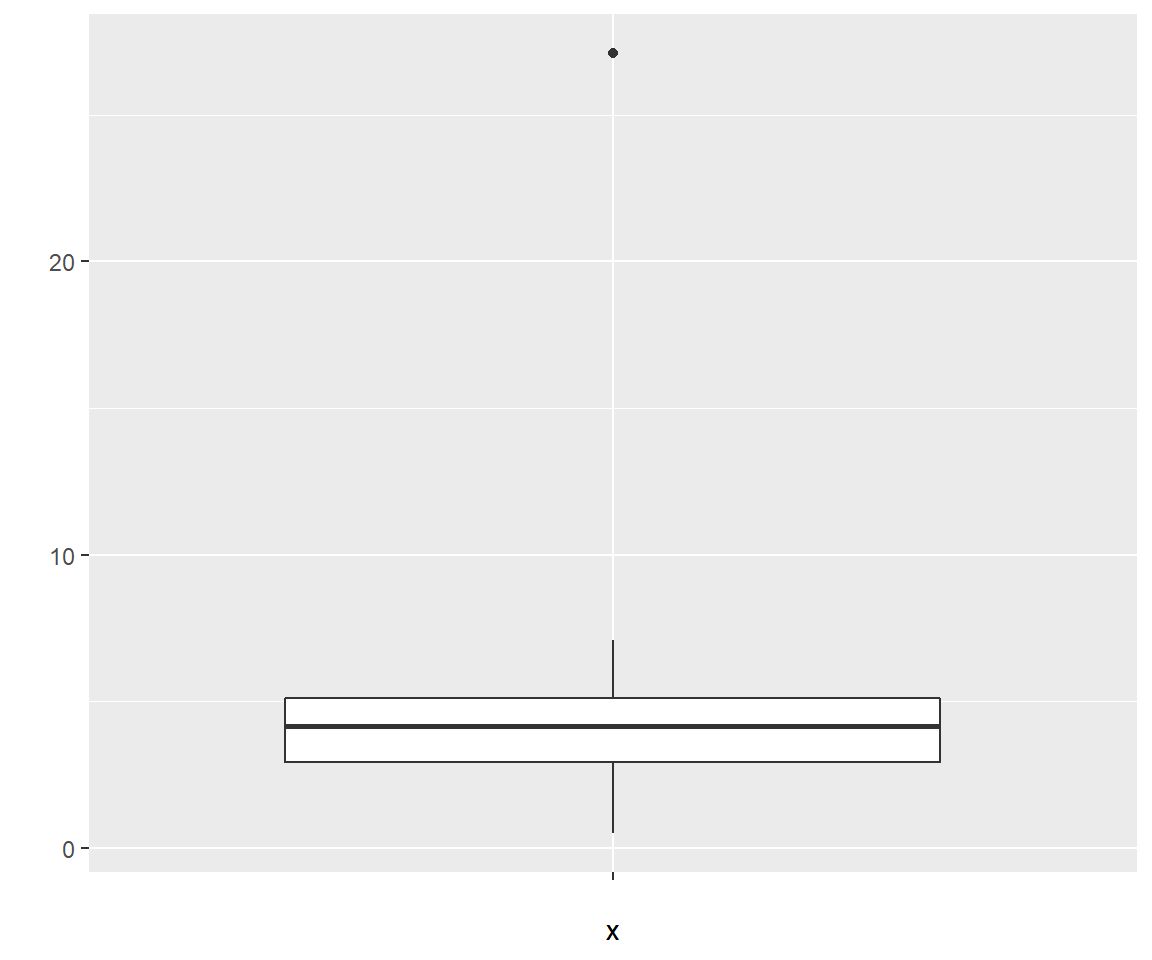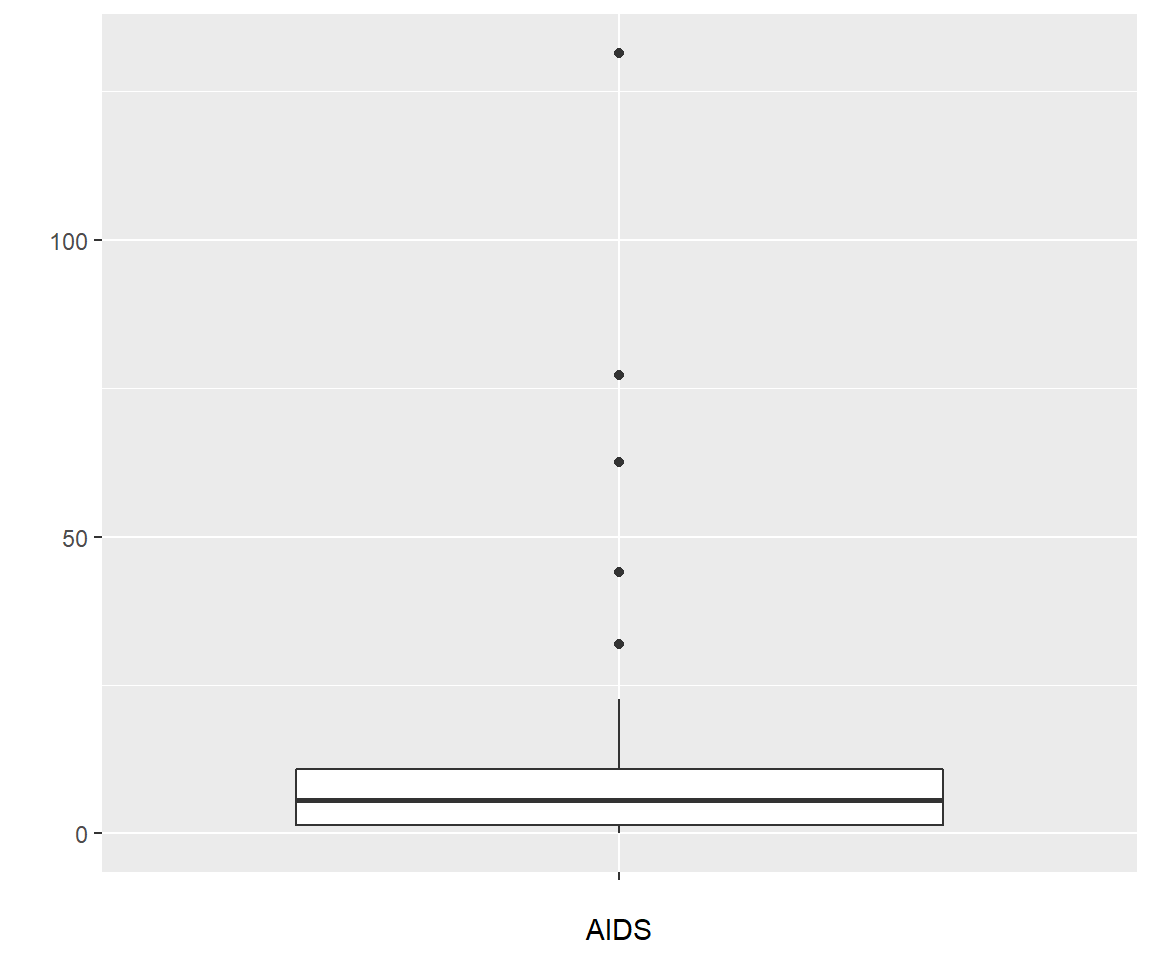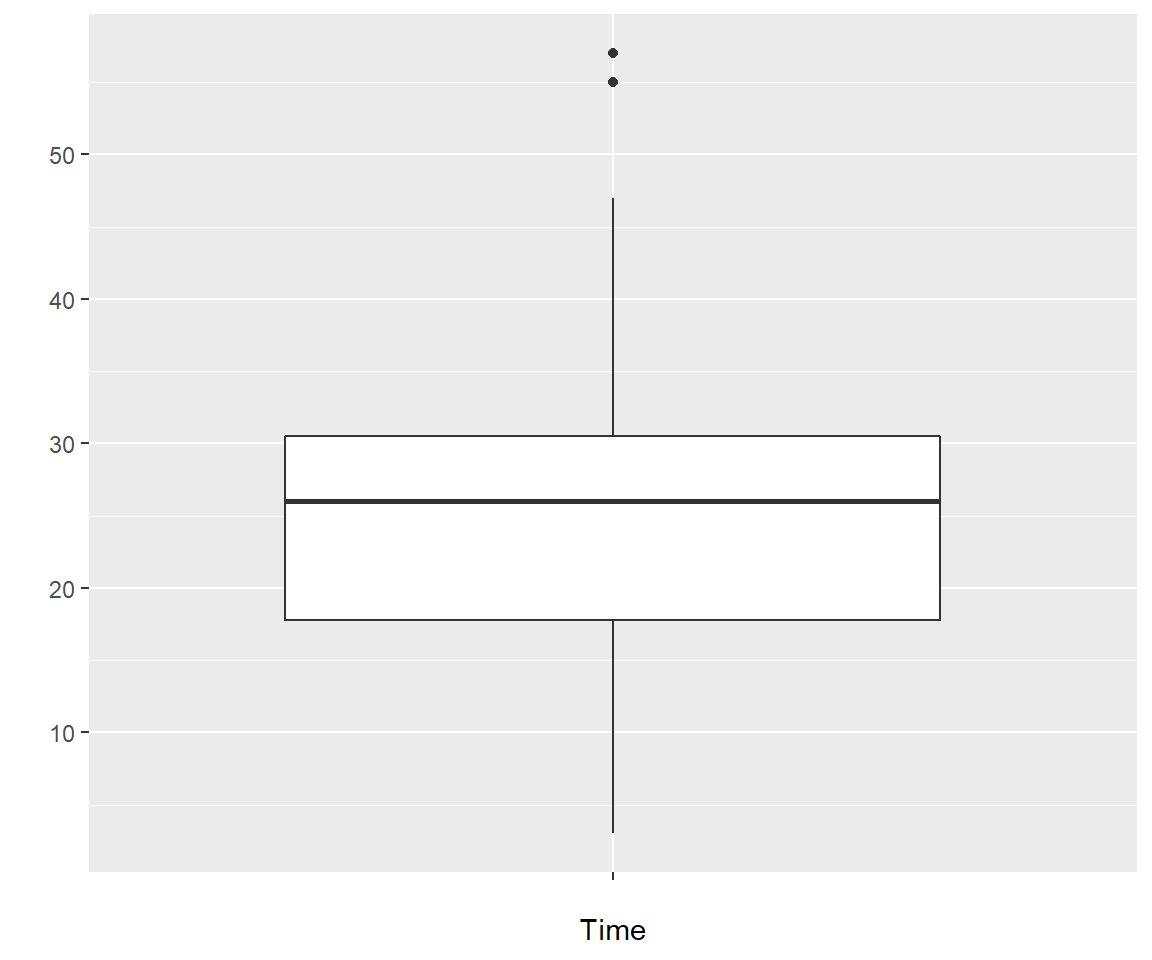## Exercises - Descriptive Statistics - Data Summaries

#### Problem 1

For each of the following variables decide whether the data is categorical or quantitative

Daily low temperature in New York

Brand of cereal in supermarket

Telephone number

Weight lost in a weight loss program

Time spent on studying for the class during last week

#### Problem 2

0.5 , 1 , 1.5 , 1.9 , 2.1 , 2.2 , 2.7 , 2.8 , 3.3 , 3.6 , 3.9 , 3.9 , 3.9 , 4 , 4 , 4.3 , 4.3 , 4.5 , 4.5 , 5 , 5 , 5.1 , 5.1 , 5.5 , 5.6 , 5.9 , 6.2 , 6.3 , 7.1 , 27.1

1. Find the mean, median, range and standard deviation.

2. Find the 20th and the 64th percentile of this data set.

3. draw the boxplot for this data set

#### Problem 3

Using the data from problem 2, find the z score of x = 3.6. What x would have a z score = 1?

#### Problem 4

Consider the data set for Friday the 13th. This data set has several comparisons of a Friday the 13th and the previous Friday the 6th, for example the number of cars passing through a junction (traffic), shoppers for a supermarket (shopping), or admissions due to transport accidents (accident)

head(friday13)
##   Dataset    six thirteen
## 1 traffic 139246   138548
## 2 traffic 134012   132908
## 3 traffic 137055   136018
## 4 traffic 133732   131843
## 5 traffic 123552   121641
## 6 traffic 121139   118723

Use R to compute the mean and the standard deviation for the two Fridays and the three data sets separately (so there will be the mean and st. dev. for the number of accidents on Friday the 6th, the mean and st. dev. for the number of accidents on Friday the 13th, the mean and st. dev. for the number of shoppers on Friday the 6th and so on).

Does any of these numbers support the idea that Friday the 13th is special?

#### Problem 5

Consider the data in AIDS in Americas in 1995.

head(aids)
##           Country  AIDS
## 1        Anguilla   0.0
## 2 Antigua Barbuda   7.3
## 3       Argentina   5.6
## 4         Bahamas 131.4
## 6          Belize   4.5
1. Find the 20th and the 80th percentiles of the AIDS rates.

2. Find the 5 number summary for the aids rates.

3. According to the boxplot, which countries are outliers in this data set?

4. Let’s say the WHO wants to use the “average” rate of AIDS infection (together with the number of people living in the Americas) to estimate the number of AIDS infected people in the Americas. Should they use the mean or the median to find the “average”?

#### Problem 6

In this exercise we study the data set

head(headache)
##   Time Dose Sex BP.Quan
## 1   35    2   0    0.25
## 2   43    2   0    0.50
## 3   55    2   0    0.75
## 4   47    2   1    0.25
## 5   43    2   1    0.50
## 6   57    2   1    0.75
1. What is the type of data of the variables?

2. Find the mean and standard deviation of Time.

3. Find the 5-number summary and draw the boxplot of Time

#### Problem 7

Company XYZ has a contract with a supplier for metal rods. The contract says that all the rods have to be between 15.26cm and 15.47cm long. XYZ just received a shipment of 50000 rods. They randomly select 100 of them and measure the length of each. They find $$\overline{X}=15.344$$ and $$s=0.041$$. Should they except this shipment?

#### Problem 8

Consider the following data set:

x <- 1:10
y <- c(3,    0,  0,  10,     8,  4,  5,  8,  14,     9)
kable(data.frame(x=x,y=y))
x y
1 3
2 0
3 0
4 10
5 8
6 4
7 5
8 8
9 14
10 9

Now we find

round(cor(x, y), 3)
##  0.704

Find another observation (a, b) such that

round(cor(c(x, a), c(y, b)), 3)
##  0

### Solutions

#### Problem 1

For each of the following variables decide whether the data is categorical or quantitative

Daily low temperature in New York - quantitative

Brand of cereal in supermarket - categorical

Telephone number - categorical

License plates of cars - categorical

Weight lost in a weight loss program - quantitative

Time spent on studying for the class during last week - quantitative

#### Problem 2

0.5 , 1 , 1.5 , 1.9 , 2.1 , 2.2 , 2.7 , 2.8 , 3.3 , 3.6 , 3.9 , 3.9 , 3.9 , 4 , 4 , 4.3 , 4.3 , 4.5 , 4.5 , 5 , 5 , 5.1 , 5.1 , 5.5 , 5.6 , 5.9 , 6.2 , 6.3 , 7.1 , 27.1

the data is comma delimited, so after copying it in R type

x <- getx(sep=",")
mean(x)
##  4.76
median(x)
##  4.15
sd(x)
##  4.51859
1. Find the 20th and the 64th percentile of this data set.
quantile(x, c(0.2, 0.64))
##  20%  64%
## 2.60 4.78
1. draw the boxplot for this data set
bplot(x)#### Problem 3

Using the data from problem 2, find the z score of x = 3.6. What x would have a z score = 1?

We found $$\overline{X}=4.76$$ and $$s= 4.519$$, so the z score of x=3.6 is
$$z = (x-\overline{X})/s = (3.6-4.76)/4.519 = -0.2567$$

We want z score =1, so

$$1 = (x-\overline{X})/s$$

or

$$s= x-\overline{X}$$

or

$$x = s+\overline{X} = 4.519+4.76 = 9.279$$

#### Problem 4

Consider the data set for Friday the 13th. This data set has several comparisons of a Friday the 13th and the previous Friday the 6th, for example the number of cars passing through a junction (traffic), shoppers for a supermarket (shopping), or admissions due to transport accidents (accident)

Use R to compute the mean and the standard deviation for the two Fridays and the three data sets separately (so there will be the mean and st. dev. for the number of accidents on Friday the 6th, the mean and st. dev. for the number of accidents on Friday the 13th, the mean and st. dev. for the number of shoppers on Friday the 6th and so on). Does any of these numbers support the idea that Friday the 13th is special?

attach(friday13)
mean(friday13[Dataset=="accident",3])
##  10.83333
mean(friday13[Dataset=="accident",3])
##  10.83333
mean(friday13[Dataset == "traffic", 2])
##  128385.3
sd(friday13[Dataset == "traffic", 2])
##  7259.223
mean(friday13[Dataset == "traffic", 3])
##  126549.5
sd(friday13[Dataset == "traffic", 3])
##  7664.282
mean(friday13[Dataset == "shopping", 2])
##  4970.511
sd(friday13[Dataset == "shopping", 2])
##  1165.615
mean(friday13[Dataset == "accident", 2])
##  7.5
sd(friday13[Dataset == "accident", 2])
##  3.331666
mean(friday13[Dataset == "accident", 3])
##  10.83333
sd(friday13[Dataset == "accident", 3])
##  3.600926

There does not appear to be anything special about Friday the 13th

#### Problem 5

Consider the data in AIDS in Americas in 1995.

1. Find the 20th and the 80th percentiles of the AIDS rates.
attach(aids)
quantile(AIDS, c(0.2,0.8))
##   20%   80%
##  0.96 13.82
1. Find the 5 number summary for the aids rates.
fivenumber(AIDS)
##  Minimum  Q1 Median   Q3 Maximum
##        0 1.5    5.6 10.9   131.4
## IQR =  9.4
1. According to the boxplot, which countries are outliers in this data set?
bplot(AIDS)the 5 countries with rates over 30are outliers, so

aids[AIDS > 30,]
##          Country  AIDS
## 4        Bahamas 131.4
## 7        Bermuda  77.2
## 21 French Guiana  62.5
## 23    Guadaloupe  31.9
1. Let’s say the WHO wants to use the “average” rate of AIDS infection (together with the number of people living in the Americas) to estimate the number of AIDS infected people in the Americas.

Should they use the mean or the median to find the “average”?

Mean, because the countries with the highest AIDS rates have to influence our “average”, and they don’t if we use the median.

#### Problem 6

In this exercise we study the data set headache

1. What is the type of data of the variables?

Time: quantitative
Dose: quantitative
Sex: categorical
BP Quan: categorical.

1. Find the mean and standard deviation of Time.
attach(headache)
mean(Time)
##  26.33333
sd(Time)
##  14.56818
1. Find the 5-number summary and draw the boxplot of Time
fivenumber(Time)
##  Minimum   Q1 Median   Q3 Maximum
##        3 17.8     26 30.5      57
## IQR =  12.7
 bplot(y=Time)#### Problem 7

Company XYZ has a contract with a supplier for metal rods. The contract says that all the rods have to be between 15.26cm and 15.47cm long. XYZ just received a shipment of 50000 rods. They randomly select 100 of them and measure the length of each. They find $$\overline{X}=15.344$$ and $$s=0.041$$. Should they except this shipment?

We can use the empirical rule to decide. This requires that the lengths of the rods have a bell-shaped histogram, which of course should be checked. Then

$$\overline{X} \pm 2s = 15.344 \pm 2 \times 0.041 = (15.262, 15.426)$$

The interval is supposed to include 95% the observations (or lengths of the rods), so we can conclude that 95% the rods have a length at least 15.262cm and at most 15.462cm, which is in accordance with the contract. So XYZ should accept the shipment.

#### Problem 8

Consider the following data set:

x <- 1:10
y <- c(3,    0,  0,  10,     8,  4,  5,  8,  14,     9)
kable(data.frame(x=x,y=y))
x y
1 3
2 0
3 0
4 10
5 8
6 4
7 5
8 8
9 14
10 9
Now w e find
round(cor(x,y), 3)

Find another observation (a, b) such that

round(cor( c(x,a), c(y,b)), 3)

There are many solutions, here is one of them:

round(cor(c(x, 19.9), c(y, -0.5)), 3)
##  0